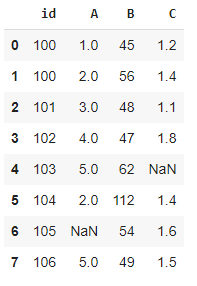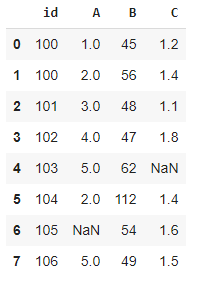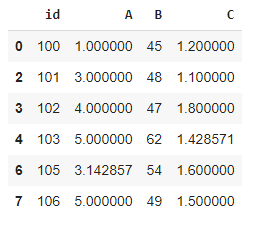# A Better Way for Data Preprocessing: Pandas Pipe

Real-life data is usually messy. It requires a lot of preprocessing to be ready for use. Pandas being one of the most-widely used data analysis and manipulation libraries offers several functions to preprocess the raw data.

In this article, we will focus on one particular function that organizes multiple preprocessing operations into a single one: the pipe function.

When it comes to software tools and packages, I learn best by working through examples. I keep this in mind when creating content. I will do the same in this article.

```import numpy as np
import pandas as pd
df = pd.DataFrame({
"id": [100, 100, 101, 102, 103, 104, 105, 106],
"A": [1, 2, 3, 4, 5, 2, np.nan, 5],
"B": [45, 56, 48, 47, 62, 112, 54, 49],
"C": [1.2, 1.4, 1.1, 1.8, np.nan, 1.4, 1.6, 1.5]
})
df```(image by author)

Our data frame contains some missing values indicated by a standard missing value representation (i.e. NaN). The id column includes duplicate values. Last but not least, 112 in column B seems like an outlier.

These are some of the typical issues in real-life data. We will be creating a pipe that handles the issues we have just described.

For each task, we need a function. Thus, the first step is to create the functions that will be placed in the pipe.

It is important to note that the functions used in the pipe need to take a data frame as argument and return a data frame.

The first function handles the missing values.

```def fill_missing_values(df):
for col in df.select_dtypes(include= ["int","float"]).columns:
val = df[col].mean()
df[col].fillna(val, inplace=True)
return df```

I prefer to replace the missing values in the numerical columns with the mean value of the column. Feel free to customize this function. It will work in the pipe as long as it takes a data frame as argument and returns a data frame.

The second function will help us remove the duplicate values.

```def drop_duplicates(df, column_name):
df = df.drop_duplicates(subset=column_name)
return df```

I have got some help from the built-in drop duplicates function of Pandas. It eliminates the duplicate values in the given column or columns. In addition to the data frame, this function also takes a column name as an argument. We can pass the additional arguments to the pipe as well.

Also read: Pandas vs SQL. When Data Scientists Should Use One Over the Other

The last function in the pipe will be used for eliminating the outliers.

```def remove_outliers(df, column_list):
for col in column_list:
avg = df[col].mean()
std = df[col].std()
low = avg - 2 * std
high = avg + 2 * std
df = df[df[col].between(low, high, inclusive=True)]
return df```

What this function does is as follows:
1. It takes a data frame and a list of columns
2. For each column in the list, it calculates the mean and standard deviation
3. It calculates a lower and upper bound using the mean and standard deviation
4. It removes the values that are outside range defined by the lower and upper bound

Just like the previous functions, you can choose your own way of detecting outliers.

We now have 3 functions that handle a data preprocessing task. The next step is to create a pipe with these functions.

```df_processed = (df.
pipe(fill_missing_values).
pipe(drop_duplicates, "id").
pipe(remove_outliers, ["A","B"]))```

This pipe executes the functions in the given order. We can pass the arguments to the pipe along with the function names.

One thing to mention here is that some functions in the pipe modify the original data frame. Thus, using the pipe as indicated above will update df as well.

One option to overcome this issue is to use a copy of the original data frame in the pipe. If you do not care about keeping the original data frame as is, you can just use it in the pipe.

I will update the pipe as below:

```my_df = df.copy()
df_processed = (my_df.
pipe(fill_missing_values).
pipe(drop_duplicates, "id").
pipe(remove_outliers, ["A","B"]))```

Let’s take a look at the original and processed data frames:df (image by author)df_processed (image by author)

Conclusion

You can, of course, accomplish the same tasks by applying these functions separately. However, the pipe function offers a structured and organized way for combining several functions into a single operation.

Depending on the raw data and the tasks, the preprocessing may include more steps. You can add as many steps as you need in the pipe function. As the number of steps increase, the syntax becomes cleaner with the pipe function compared to executing functions separately.

“A Better Way for Data Preprocessing: Pandas Pipe”
– Soner Yıldırım

### Related Stories

Jan 06, 2023

#### Top 2 Online Data Science Courses to Improve your Career in 2023

The discipline of Data Science is expanding quickly and has enormous promise. It is used in various sectors, including manufacturing, retail, healt...By Nikos_DataSource
Nov 25, 2021

#### 5 Tips To Ace Your Job Interview For A Data Scientist Opening

5 Tips To Ace Your Job Interview For A Data Scientist Opening.PNG 795.94 KBImage SourceAspiring data scientists have a bright future ahead of them....By Daniel Morales
Nov 12, 2021

#### When to Avoid Deep Learning